Скачать презентацию Investment Method also known as Income Capitalization Approach

7cb15954bf15f10b3ce9ca1144092558.ppt

• Количество слайдов: 18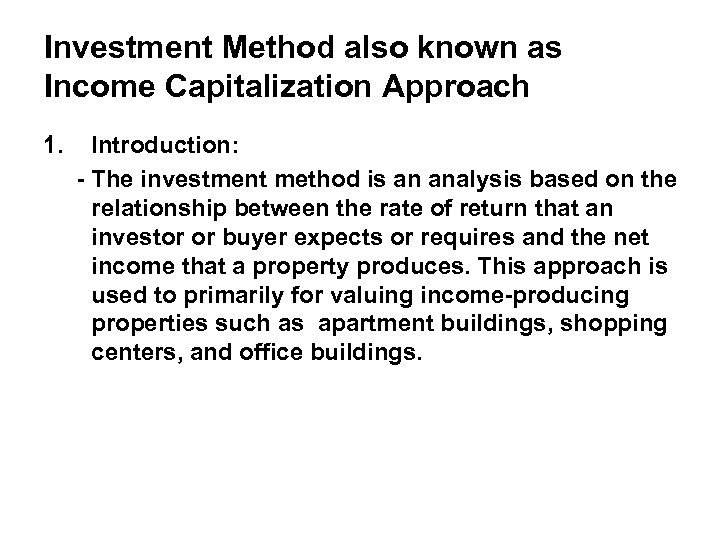Investment Method also known as Income Capitalization Approach 1. Introduction: - The investment method is an analysis based on the relationship between the rate of return that an investor or buyer expects or requires and the net income that a property produces. This approach is used to primarily for valuing income-producing properties such as apartment buildings, shopping centers, and office buildings.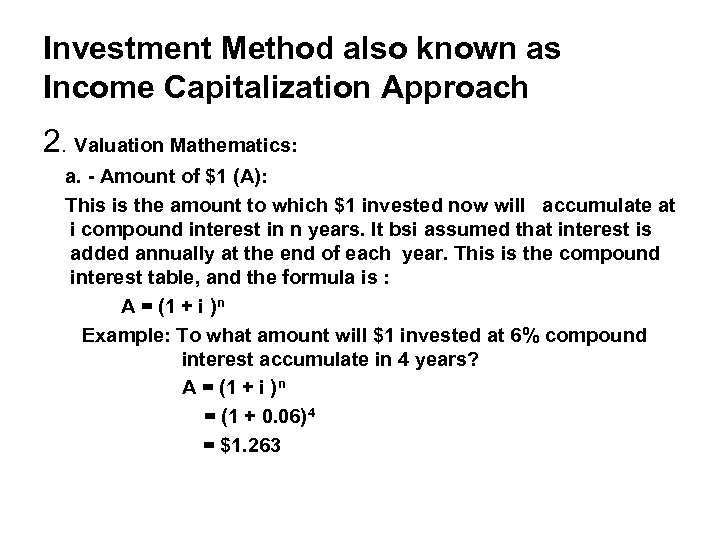Investment Method also known as Income Capitalization Approach 2. Valuation Mathematics: a. - Amount of \$1 (A): This is the amount to which \$1 invested now will accumulate at i compound interest in n years. It bsi assumed that interest is added annually at the end of each year. This is the compound interest table, and the formula is : A = (1 + i )n Example: To what amount will \$1 invested at 6% compound interest accumulate in 4 years? A = (1 + i )n = (1 + 0. 06) 4 = \$1. 263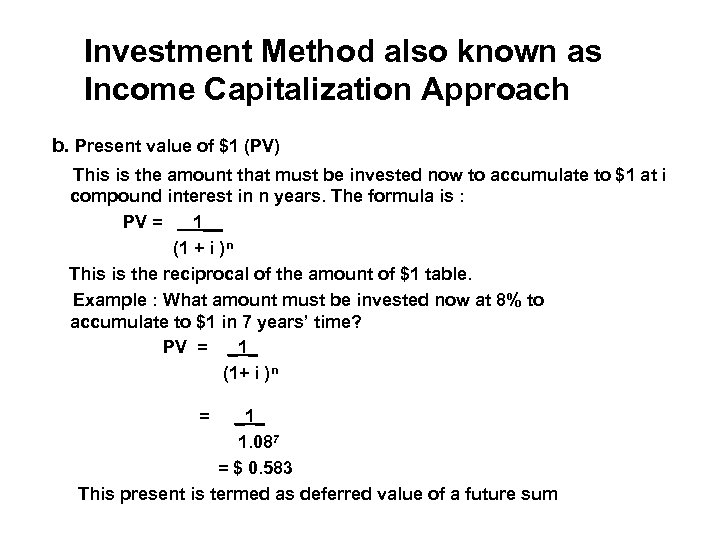Investment Method also known as Income Capitalization Approach b. Present value of \$1 (PV) This is the amount that must be invested now to accumulate to \$1 at i compound interest in n years. The formula is : PV = 1__ (1 + i )n This is the reciprocal of the amount of \$1 table. Example : What amount must be invested now at 8% to accumulate to \$1 in 7 years’ time? PV = _1_ (1+ i ) n = _1_ 1. 08 7 = \$ 0. 583 This present is termed as deferred value of a future sum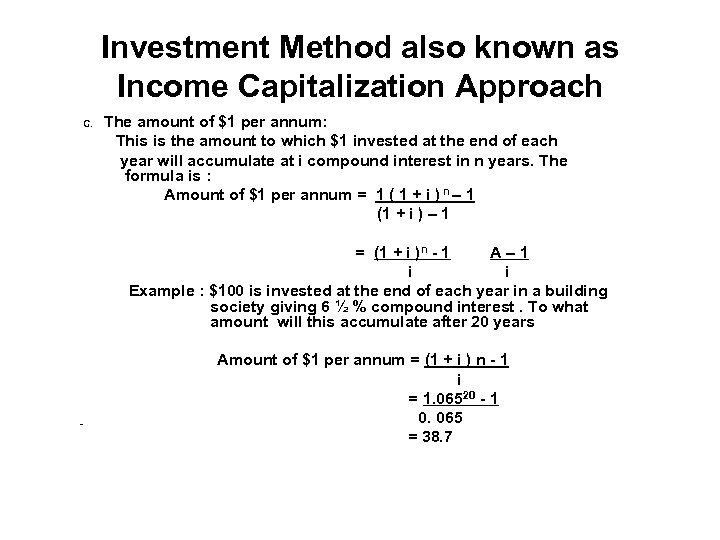Investment Method also known as Income Capitalization Approach C. The amount of \$1 per annum: This is the amount to which \$1 invested at the end of each year will accumulate at i compound interest in n years. The formula is : Amount of \$1 per annum = 1 ( 1 + i ) n – 1 (1 + i ) – 1 = (1 + i )n - 1 A– 1 i i Example : \$100 is invested at the end of each year in a building society giving 6 ½ % compound interest. To what amount will this accumulate after 20 years Amount of \$1 per annum = (1 + i ) n - 1 i = 1. 06520 - 1 0. 065 = 38. 7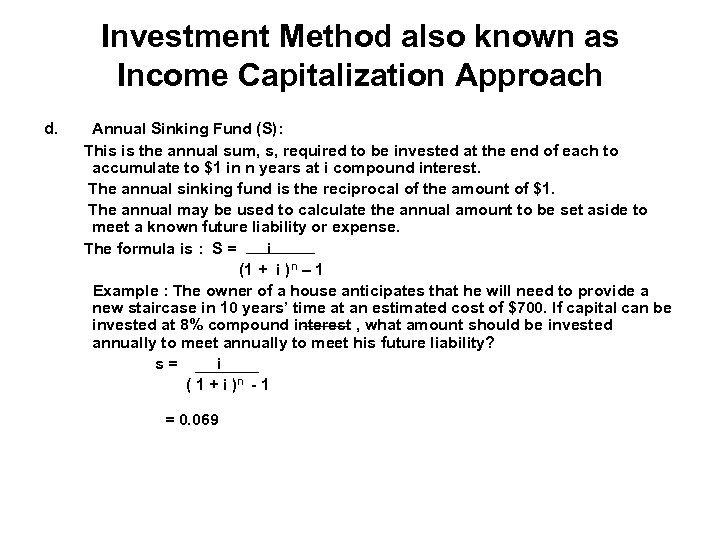Investment Method also known as Income Capitalization Approach d. Annual Sinking Fund (S): This is the annual sum, s, required to be invested at the end of each to accumulate to \$1 in n years at i compound interest. The annual sinking fund is the reciprocal of the amount of \$1. The annual may be used to calculate the annual amount to be set aside to meet a known future liability or expense. The formula is : S = i (1 + i ) n – 1 Example : The owner of a house anticipates that he will need to provide a new staircase in 10 years’ time at an estimated cost of \$700. If capital can be invested at 8% compound interest , what amount should be invested annually to meet his future liability? s= i ( 1 + i )n - 1 = 0. 069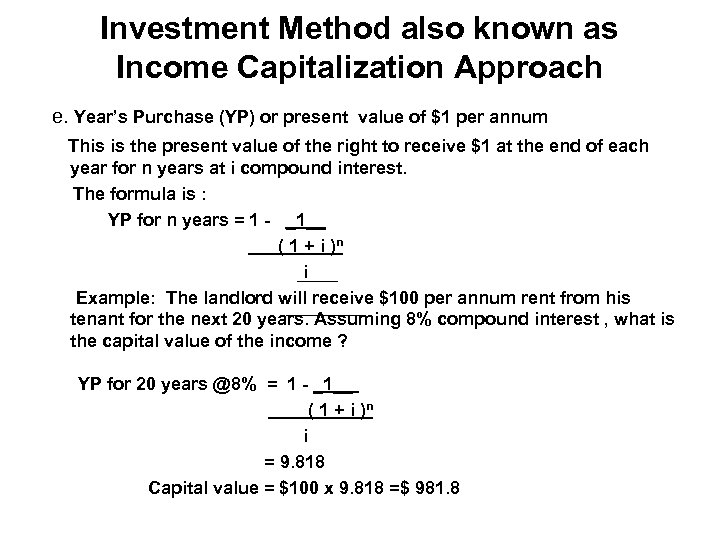Investment Method also known as Income Capitalization Approach e. Year’s Purchase (YP) or present value of \$1 per annum This is the present value of the right to receive \$1 at the end of each year for n years at i compound interest. The formula is : YP for n years = 1 - _1__ ( 1 + i )n i Example: The landlord will receive \$100 per annum rent from his tenant for the next 20 years. Assuming 8% compound interest , what is the capital value of the income ? YP for 20 years @8% = 1 - _1__ ( 1 + i )n i = 9. 818 Capital value = \$100 x 9. 818 =\$ 981. 8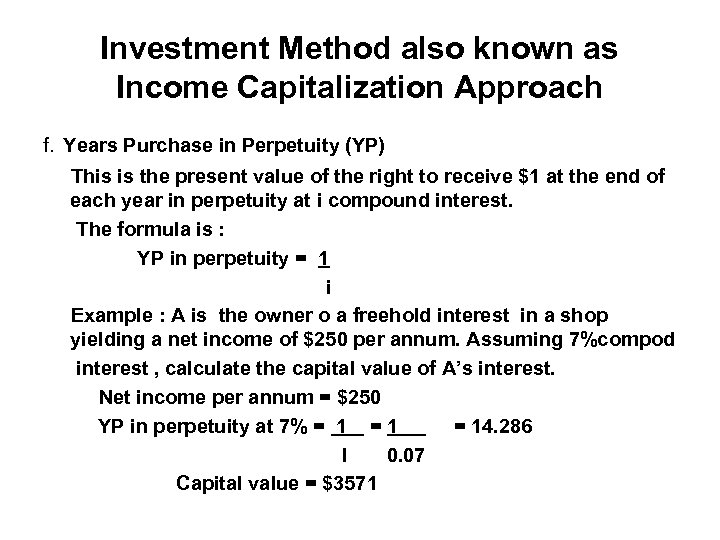Investment Method also known as Income Capitalization Approach f. Years Purchase in Perpetuity (YP) This is the present value of the right to receive \$1 at the end of each year in perpetuity at i compound interest. The formula is : YP in perpetuity = 1 i Example : A is the owner o a freehold interest in a shop yielding a net income of \$250 per annum. Assuming 7%compod interest , calculate the capital value of A’s interest. Net income per annum = \$250 YP in perpetuity at 7% = 1 = 14. 286 I 0. 07 Capital value = \$3571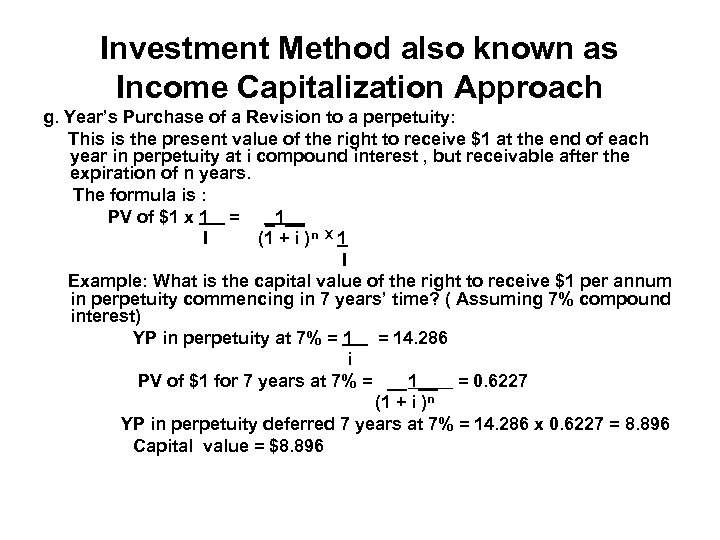Investment Method also known as Income Capitalization Approach g. Year’s Purchase of a Revision to a perpetuity: This is the present value of the right to receive \$1 at the end of each year in perpetuity at i compound interest , but receivable after the expiration of n years. The formula is : PV of \$1 x 1 = _1__ I (1 + i ) n X 1 I Example: What is the capital value of the right to receive \$1 per annum in perpetuity commencing in 7 years’ time? ( Assuming 7% compound interest) YP in perpetuity at 7% = 14. 286 i PV of \$1 for 7 years at 7% = __1__ = 0. 6227 (1 + i )n YP in perpetuity deferred 7 years at 7% = 14. 286 x 0. 6227 = 8. 896 Capital value = \$8. 896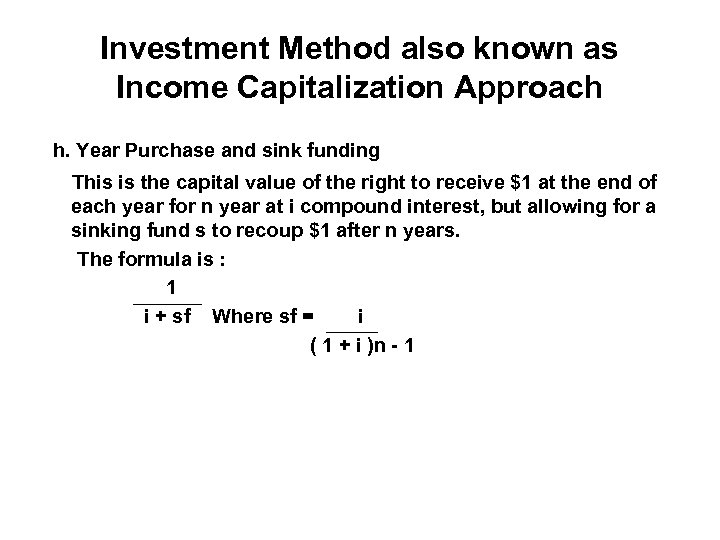Investment Method also known as Income Capitalization Approach h. Year Purchase and sink funding This is the capital value of the right to receive \$1 at the end of each year for n year at i compound interest, but allowing for a sinking fund s to recoup \$1 after n years. The formula is : 1 i + sf Where sf = i ( 1 + i )n - 1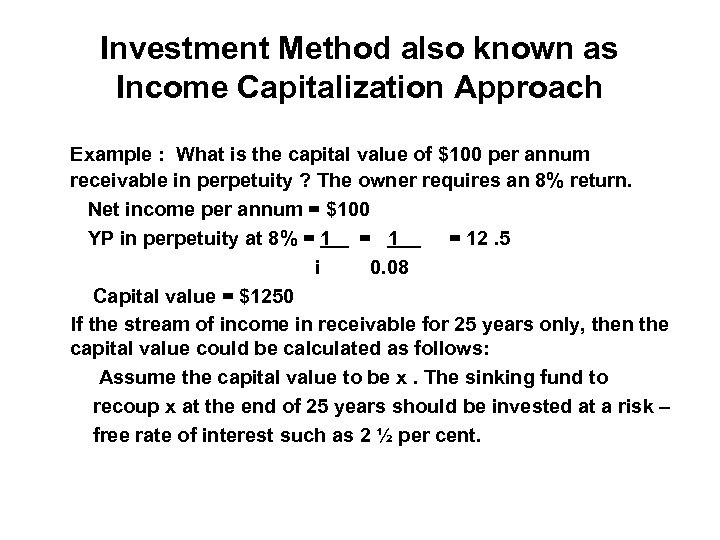Investment Method also known as Income Capitalization Approach Example : What is the capital value of \$100 per annum receivable in perpetuity ? The owner requires an 8% return. Net income per annum = \$100 YP in perpetuity at 8% = 1 = 12. 5 i 0. 08 Capital value = \$1250 If the stream of income in receivable for 25 years only, then the capital value could be calculated as follows: Assume the capital value to be x. The sinking fund to recoup x at the end of 25 years should be invested at a risk – free rate of interest such as 2 ½ per cent.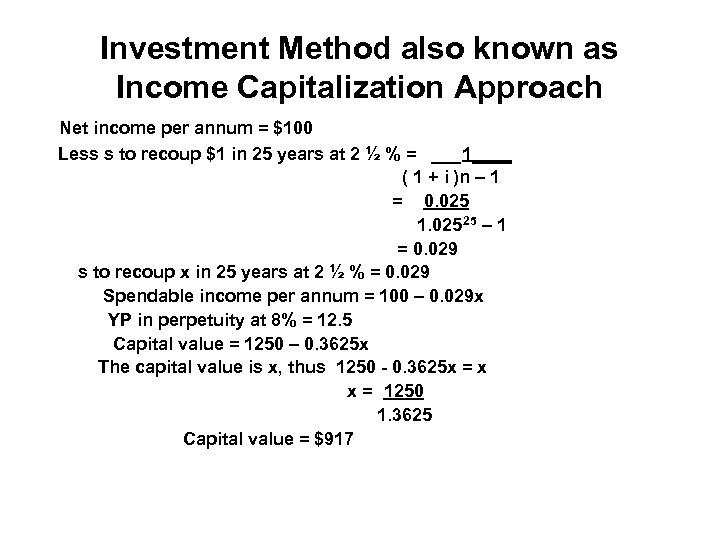Investment Method also known as Income Capitalization Approach Net income per annum = \$100 Less s to recoup \$1 in 25 years at 2 ½ % = ___1____ ( 1 + i )n – 1 = 0. 025 1. 025 25 – 1 = 0. 029 s to recoup x in 25 years at 2 ½ % = 0. 029 Spendable income per annum = 100 – 0. 029 x YP in perpetuity at 8% = 12. 5 Capital value = 1250 – 0. 3625 x The capital value is x, thus 1250 - 0. 3625 x = x x = 1250 1. 3625 Capital value = \$917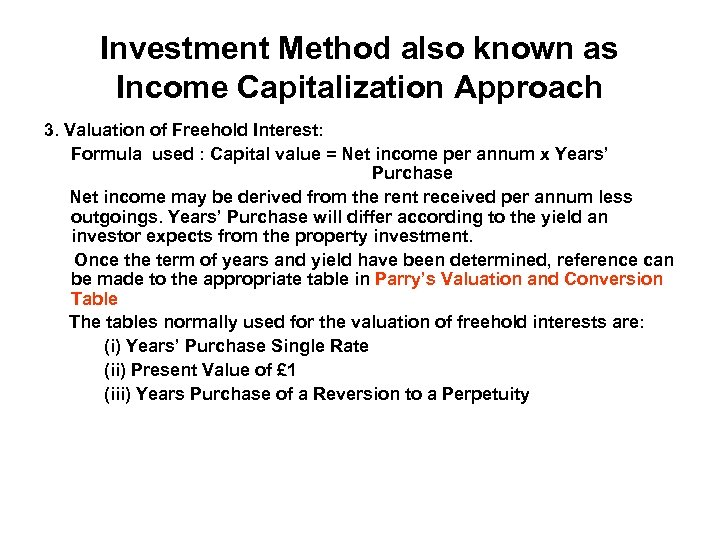Investment Method also known as Income Capitalization Approach 3. Valuation of Freehold Interest: Formula used : Capital value = Net income per annum x Years’ Purchase Net income may be derived from the rent received per annum less outgoings. Years’ Purchase will differ according to the yield an investor expects from the property investment. Once the term of years and yield have been determined, reference can be made to the appropriate table in Parry’s Valuation and Conversion Table The tables normally used for the valuation of freehold interests are: (i) Years’ Purchase Single Rate (ii) Present Value of £ 1 (iii) Years Purchase of a Reversion to a Perpetuity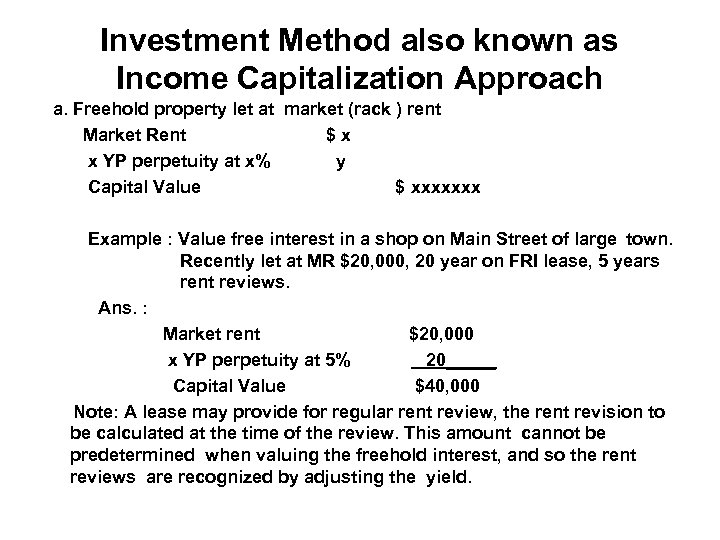Investment Method also known as Income Capitalization Approach a. Freehold property let at market (rack ) rent Market Rent \$x x YP perpetuity at x% y Capital Value \$ xxxxxxx Example : Value free interest in a shop on Main Street of large town. Recently let at MR \$20, 000, 20 year on FRI lease, 5 years rent reviews. Ans. : Market rent \$20, 000 x YP perpetuity at 5% 20_____ Capital Value \$40, 000 Note: A lease may provide for regular rent review, the rent revision to be calculated at the time of the review. This amount cannot be predetermined when valuing the freehold interest, and so the rent reviews are recognized by adjusting the yield.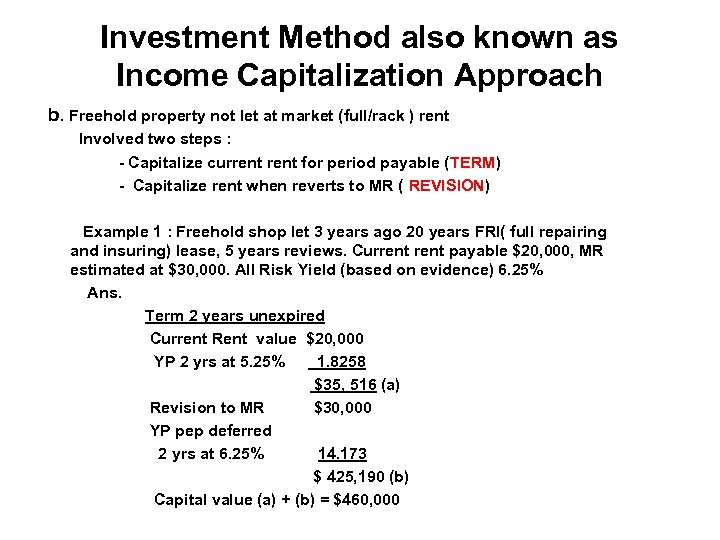Investment Method also known as Income Capitalization Approach b. Freehold property not let at market (full/rack ) rent Involved two steps : - Capitalize current for period payable (TERM) - Capitalize rent when reverts to MR ( REVISION) Example 1 : Freehold shop let 3 years ago 20 years FRI( full repairing and insuring) lease, 5 years reviews. Current payable \$20, 000, MR estimated at \$30, 000. All Risk Yield (based on evidence) 6. 25% Ans. Term 2 years unexpired Current Rent value \$20, 000 YP 2 yrs at 5. 25% 1. 8258 \$35, 516 (a) Revision to MR \$30, 000 YP pep deferred 2 yrs at 6. 25% 14. 173 \$ 425, 190 (b) Capital value (a) + (b) = \$460, 000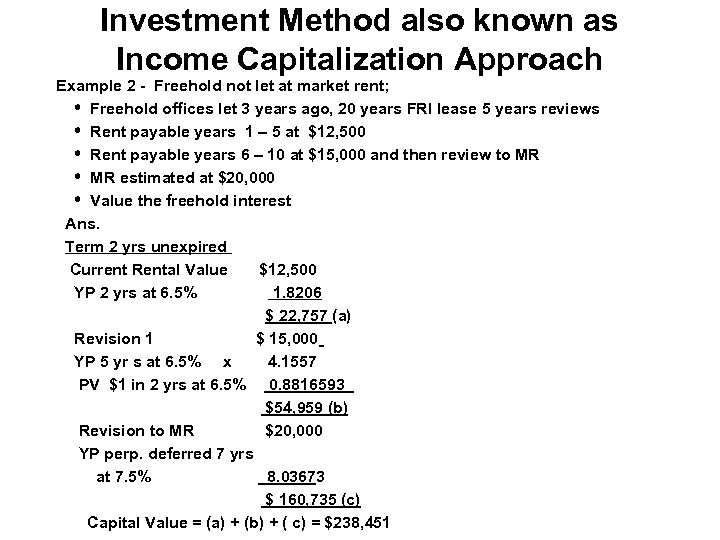Investment Method also known as Income Capitalization Approach Example 2 - Freehold not let at market rent; Freehold offices let 3 years ago, 20 years FRI lease 5 years reviews Rent payable years 1 – 5 at \$12, 500 Rent payable years 6 – 10 at \$15, 000 and then review to MR estimated at \$20, 000 Value the freehold interest Ans. Term 2 yrs unexpired Current Rental Value \$12, 500 YP 2 yrs at 6. 5% 1. 8206 \$ 22, 757 (a) Revision 1 \$ 15, 000 YP 5 yr s at 6. 5% x 4. 1557 PV \$1 in 2 yrs at 6. 5% 0. 8816593 \$54, 959 (b) Revision to MR \$20, 000 YP perp. deferred 7 yrs at 7. 5% 8. 03673 \$ 160, 735 (c) Capital Value = (a) + (b) + ( c) = \$238, 451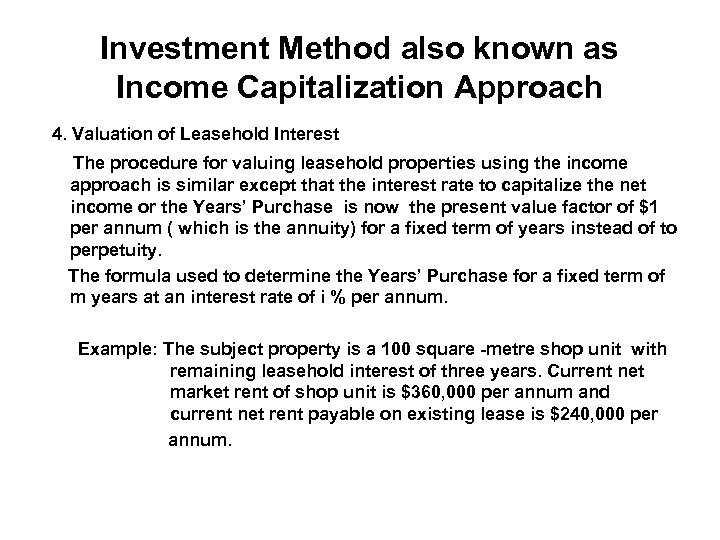Investment Method also known as Income Capitalization Approach 4. Valuation of Leasehold Interest The procedure for valuing leasehold properties using the income approach is similar except that the interest rate to capitalize the net income or the Years’ Purchase is now the present value factor of \$1 per annum ( which is the annuity) for a fixed term of years instead of to perpetuity. The formula used to determine the Years’ Purchase for a fixed term of m years at an interest rate of i % per annum. Example: The subject property is a 100 square -metre shop unit with remaining leasehold interest of three years. Current net market rent of shop unit is \$360, 000 per annum and current net rent payable on existing lease is \$240, 000 per annum.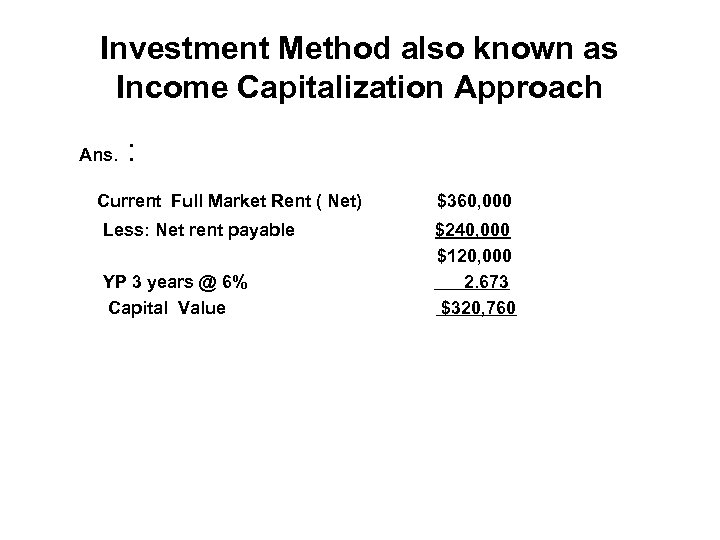Investment Method also known as Income Capitalization Approach Ans. : Current Full Market Rent ( Net) Less: Net rent payable YP 3 years @ 6% Capital Value \$360, 000 \$240, 000 \$120, 000 2. 673 \$320, 760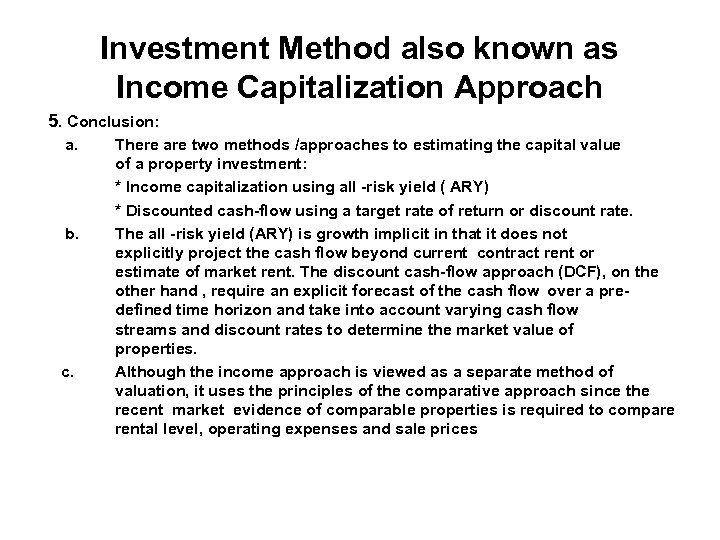Investment Method also known as Income Capitalization Approach 5. Conclusion: a. b. c. There are two methods /approaches to estimating the capital value of a property investment: * Income capitalization using all -risk yield ( ARY) * Discounted cash-flow using a target rate of return or discount rate. The all -risk yield (ARY) is growth implicit in that it does not explicitly project the cash flow beyond current contract rent or estimate of market rent. The discount cash-flow approach (DCF), on the other hand , require an explicit forecast of the cash flow over a predefined time horizon and take into account varying cash flow streams and discount rates to determine the market value of properties. Although the income approach is viewed as a separate method of valuation, it uses the principles of the comparative approach since the recent market evidence of comparable properties is required to compare rental level, operating expenses and sale prices